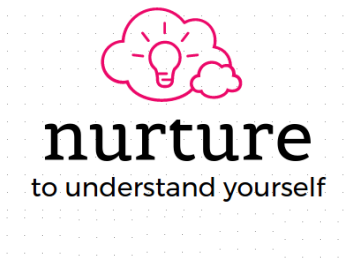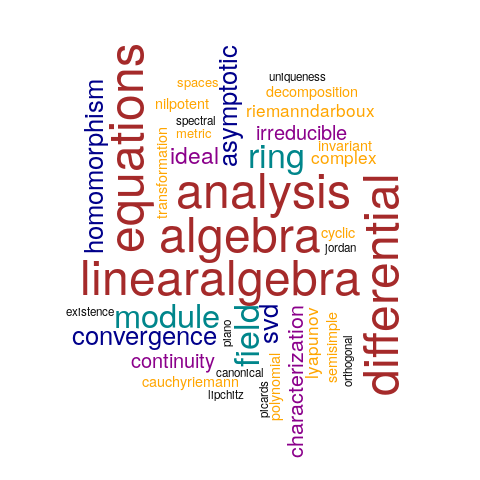nurture 2017
Learning Strategies for Postgraduates to the memory of Subrahmanyan Chandrasekhar
4th - 16th December 2017
10th - 22nd December 2017
Central University of Tamil Nadu
Funded by INSA (expected)This workshop will aim to touch upon some of these topics

Algebra

• Fields, subrings. homomorphism of rings. Kernel and image of a homomorphism. Characteristic of a ring.
• Quotient rings. Prime ideals, maximal ideals and their characterization.
• Polynomial rings. Divisibility. units. Factorization in a ring. Irreducible and prime elements in a ring.
• Unique factorization domain, principal ideal domain and euclidean domains.

Analysis

• Riemann-Darboux integral
• Pointwise and uniform convergence:
• Complex Analysis: Continuity, differentiation, analytic/holomorphic functions, Cauchy-Riemann equations,
• Introduction to metric spaces

Linear Algebra

• Structure of a single linear transformation: Invariant subspaces, semi-simple and nilpotent maps;
• Characteristic polynomial and minimal polynomial;
• Primary decomposition, cyclic subspaces and decomposition into cyclic subspaces, Jordan and rational canonical forms.
• Spectral theorem for orthogonal and unitary linear maps. Singular value decomposition.
• Modules: Definition and examples, submodules, direct sums, module homomorphisms, quotient modules, torsion elements, free modules

Differential Equations

• BVPs and greens functions, Lipchitz continuity, Lyapunov stability
• Existence and uniqueness theorem on ODE namely Picards iteration and Pieno theorem
• Solution of stiff equations using asymptotic expansion
• Introduction to numerics, Fundamental matrix solutions and exponential of matrices

Workshop Poster

Subrahmanyan Chandrasekhar
(19 Oct 1910 - 21 Aug 1995)Workshop Cloud